Reach Us+44-1235-425476
A Note on the Calculation of Intrasubject Coefficient of Variation in Bioequivalence Trails | OMICS International
Journal of Bioanalysis & Biomedicine

Like us on:

All submissions of the EM system will be redirected to Online Manuscript Submission System. Authors are requested to submit articles directly to Online Manuscript Submission System of respective journal.

# A Note on the Calculation of Intrasubject Coefficient of Variation in Bioequivalence Trails

Mahmoud Abdel Mohsen*

Pharmaceutical Research Unit (PRU), Royal Scientific Society (RSS), P.O. Box 1438, Amman 11941, Jordan

*Corresponding Author:
Dr. Mahmoud Abdel Mohsen
Division of Biometrics
Pharmaceutical Research Unit
Royal Scientific Society
P.O. Box 1438, Amman 11941, Jordan
Tel: +962-6-5358290
Fax: +962-6-5358261
E-mail: [email protected]

Received Date: July 13, 2010; Accepted Date: August 24, 2010; Published: August 24, 2010

Citation: Mohsen MA (2010) A Note on the Calculation of Intrasubject Coefficient of Variation in Bioequivalence Trails. J Bioanal Biomed 2:075-078. doi:10.4172/1948-593X.1000026

Copyright: © 2010 Mohsen MA. This is an open-access article distributed under the terms of the Creative Commons Attribution License, which permits unrestricted use, distribution, and reproduction in any medium, provided the original author and source are credited.

Visit for more related articles at Journal of Bioanalysis & Biomedicine

#### Abstract

Bioequivalence studies are generally performed as crossover studies and, therefore, information in the Intrasubject Coef fi cient of Variation (CV) is needed for sample size planning. Recently, a confusing point was noticed in calculating the Intrasubject Coef fi cient of Variation (CV) in crossover studies under the additive model (i.e. under normality assumption and the multiplicative mode (i.e under logarithmic distribution). The aim of this paper is to clarify this confusion. Methods used in calculating the Intrasubject Coef fi cient of Variation (CV) are reviewed in this paper from a statistical point of view.

#### Keywords

Coefficient of variation; Bioequivalence; Crossover design; Sample size; Normal and log-normal distribution

#### Introduction

Bioavailability (BA) and Bioequivalence (BE) studies investigate and compare pharmacological characteristics of different drug formulations in terms of the rate and the extent of absorption of active ingredients.

The most common pharmacokinetic responses of the rate and the extent of absorption are: Area under the plasma concentration-time curve (AUC), maximum plasma concentration (Cmax), and time to reach maximum plasma concentration (tmax).

The distributions of responses such as AUC and Cmax are often positively skewed and exhibit a lack of homogeneity of variances. Therefore, normality assumption on AUC and Cmax may not be suitable. In this case, the assessment of average BE based on raw data and normality assumptions may not be appropriate. Logarithmic transformation is the commonly chosen data transformation technique for BE studies and is also recommended by the FDA “Guidance on Statistical Procedures for Bioequivalence Studies using a Standard Two-Treatment Crossover Design (July 1992)”.

The most important variation in crossover designs is the intrasubject or within-subject variance, which is the variation, exhibited by a single person when given the same dose of a drug over repeated administrations.

The magnitude of the intrasubject variance depends on the pharmacokinetics of the drug itself. For instance, drugs with simple kinetics (e.g. with little metabolism or well absorption) will generally have estimated intrasubject coefficient of variations (CVs) of less than 20%, whereas drugs with complicated kinetics (e.g. highly variable absorption) can have estimated coefficient of variations (CVs) that are equal to or greater than 40%. Therefore, information on intrasubject coefficient of variation (CV) is needed for sample size planning of future studies.

We believe that (Chow and Liu, 2000) in Chapter (6) of “Transformation and Analysis of Individual Subjects Ratios” had erroneously evaluated the Intrasubject Coefficient of Variation (CV) using a certain example. In this note, we will suggest a better formula in finding the Intrasubject (CV) under the two models of normality assumptions:

1. Additive model (raw data model).

2. Multiplicative model (log-transformed model).

The Intrasubject (CV) can be estimated from the analysis of variance of the parameters: AUC0→8, Cmax, and Cmax/ AUC0→8 based on the residual mean square for error.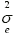Theorem (1): Let X1, X2….Xn be a sample from normal distribution, that is X ~ N (µσ2) then the coefficient of variation of X is defined as:

Coefficient of Variation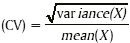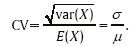In BE studies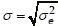,where µR is the mean of the reference formulation. Hence, the Intrasubject Coefficient of Variation (CV) under raw data normality assumption is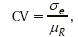Which can be estimated by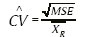(1)

Where:

MSE is the mean square error for within subject variability (not for subject within sequence error),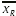is the least square (LS) mean for the reference formulation.

Multiplication of (1) by 100 gives the percent Intrasubject CV for random variable X (raw data, normality assumption).

Theorem (2): Let X1, X2, …..., Xn, be a sample from log-normal distribution; that is Ln(X) ~ N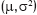. Then, the Coefficient of Variation of (X) is defined as: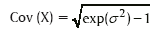Proof: Now let X1, X2….., Xn be a sample from the log-normal distribution then Y1= Ln(X1), …..Yn= Ln(Xn) is a sample from a Ndistribution.

Straight forward computation yield: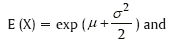Var (X) = exp (2µ,σ2) exp ((σ2) – 1),

Then by using Theorem (1), the Coefficient of Variation (CV) of X is defined as:

Coefficient of Variation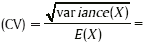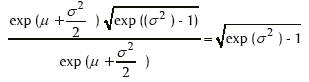In BE studies,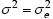, where: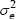is within subject variability.

Hence, the Intrasubject CV under log-transformed data, normality assumption is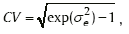This can be estimated by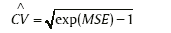(2)

Where: MSE is the mean square error for within subject variability (not for subject within sequence).

Multiplication of (2) by 100 gives the percent Intrasubject CV for a random variable X (log-transformed data, normality assumption).

#### Remark

Now we can distinguish between (formula 1) and (formula 2) in finding the Coefficient of Variation (CV) for a given random variable.

Under raw data, (formula 1) depends on two unknown parameters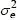and μR . On the other hand, under log-transformed data, (formula 2) depends on one unknown parameter.

Consequently, in order to build a good BE study, we recommend using the log-transformed data transformation in crossover designs.

If one is interested in finding the (1 - α) level confidence interval for the intrasubject coefficient of variation (CV) under log-normal distribution in a 2x2 crossover design, one can use the following:

(Theorem 3): Assuming a log-normal distribution and a 2x2 crossover design, it is known that a (1 - α) level confidence interval foris [ Le, Ue ].

Where: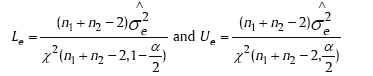Where X2(n1 + n2 − 2) denotes the cumulative distribution function of a central chi-squared distribution with (n1+n2 - 2) degrees of freedom. Thus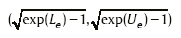(3)

is the (1 - α) level confidence interval for the intrasubject coefficient of variation (CV).

The measure of sensitivity

The index of measure of sensitivity is defined as the ratio of the width of the average BE interval (2Δ = θU – θL), to standard error of the difference in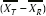least square means for the Test and the Reference formulations, that is: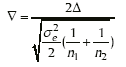1. Under raw data, normality assumption, and according to the ± 20 rule,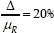, therefore, ∇ can be written in terms of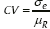as follows: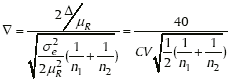(4)

(See for example in (Chow and Liu, 2000).

2. Under Log transformed data, normality assumption, and according to the equivalence limit = (Ln (0.8), Ln (1.25)), ▽ can be written in terms of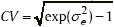as follows: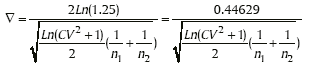(5)

In the two cases, this index (▽) is a function that depends on the Coefficient of Variation (CV) and the sample sizes; n1 and n2 used in BE studies.

(Schuirmann, 1987) used this index to compare the size of the two one-sided test procedures to the power approach.

Under raw data, normality assumption, (Chow and Liu, 2000) calculated values of ▽ for different combinations of CVs, n1, and n2 as shown in (Table 1).

Nominal concentration (ng/mL) Observed concentration
(ng/mL, mean ± S.D.)
Precision a
(%)
Accuracy b
(%)
10 10.17 ± 0.21 2.05 101.67
20 19.27 ± 1.15 5.97 96.33
50 44.35 ± 1.06 2.39 88.70
100 107.67 ± 4.04 3.75 107.67
200 216.67 ± 1.53 0.71 108.33
500 489.33 ± 20.13 4.11 97.87
1000 925.33 ± 22.19 2.40 92.53
2000 1940 ± 70 3.61 97

Table 1: Precision and accuracy data of back-calculated concentrations of calibration samples for curcumin in rat plasma (n = 3).

Under log-transformed data, normality assumption, we recalculated values of ▽ for different combinations of CVs, n1, and n2 as shown in (Table 2).

Total Sample Size (n1+n2) CV
10% 15% 20% 25% 30% 35% 40%
8 8.95 5.98 4.51 3.63 3.04 2.63 2.31
10 10.00 6.69 5.04 4.05 3.39 2.94 2.59
12 10.96 7.33 5.52 4.44 3.72 3.22 2.84
14 11.84 7.92 5.96 4.79 4.02 3.47 3.06
16 12.65 8.46 6.37 5.13 4.30 3.71 3.28
18 13.42 8.98 6.76 5.44 4.56 3.94 3.48
20 14.15 9.46 7.13 5.73 4.81 4.15 3.66
22 14.84 9.92 7.47 6.01 5.04 4.35 3.84
24 15.49 10.36 7.81 6.28 5.27 4.55 4.01

Table 2: Values of the Index of Sensitivity.

From the two (Tables 1 and 2), the results indicate that▽ increases as sample size increases and as CV decreases.

(Chow and Liu, 2000) in (Chapter 6) of “Transformation and Analysis of Individual Subjects Ratios”, considered the following example from (Clayton and Leslie, 1981).

Two Erythromycin formulations were compared using the towsquare, two-period, two-treatment crossover design involving 18 healthy subjects. The two formulations were:

T: Erythromycin stearate [Erythrocin®, 500 mg Ovaloid Tablets, 6316, Abbott Australasia pty. Ltd.], and

R: Erythromycin base [Eryc®, 2X250 mg Capsules, containing enteric-coated pellets, F.H Faulding & Co. Ltd.].

The study technique of the analysis of variance was used to test for treatment differences. However, (Clayton and Leslie, 1981) did not provide the information for the sequence assignment of subjects. For the purpose of illustration, we will adapt the order of periods and sequences from (Chow and Liu, 2000).

Nine subjects were randomized to sequence (TR) (1-9), while the remaining nine subjects were randomized to sequence (RT) (10-18). A one-week washout period separated the two periods. Blood samples were taken just before drug administration and then at: 0.50, 1.00, 1.50, 2.00, 3.00, 4.00, 6.00, and 8.00 hours post-dosing. The primary summary variables of interest, were AUC(0-8) and Cmax. The data are given in the tables, Bioequivalence data set from (Clayton and Leslie, 1981).

The results of the Analysis of Variance (ANOVA) on log-transformed and untransformed data are given in (Tables 4, 5, 6, and 7) for the two parameters; AUC(0-8) and Cmax.

Sequence Subject Period I Period II
AUC Cmax AUC Cmax
TR 1 2.52 1.04 5.47 1.58
2 8.87 3.80 4.48 1.35
3 0.79 0.12 2.25 0.77
4 1.68 0.62 1.82 1.23
5 6.95 2.92 7.87 2.25
6 1.05 0.16 3.25 0.85
7 0.99 0.28 12.39 3.00
8 5.60 2.62 4.77 2.05
9 3.16 1.51 1.88 0.60
RT 10 4.98 1.61 3.19 1.37
11 7.14 1.98 9.83 3.74
12 1.81 0.56 2.91 1.16
13 7.34 2.40 4.58 1.79
14 4.25 1.04 7.05 2.51
15 6.66 2.67 3.41 1.55
16 4.76 1.47 2.49 0.82
17 7.16 1.90 6.18 2.57
18 5.52 2.47 2.85 1.07

Table 3

Source D.F SS MS F-value p-value
Subjects 17 9.64872 0.56757 1.8368 0.11543
Sequence 1 1.30529 1.30529 2.5031 0.13319
Subject (sequence) 16 8.34343 0.52146 1.6876 0.15275
Periods 1 0.19586 0.19586 0.6338 0.43762
Treatments 1 1.04699 1.04699 3.3883 0.08428
Residual 16 4.94398 0.30899 - -
Total 35 15.83555

Table 4: Analysis of Variance for in (AUC(0-8)).

Source D.F SS MS F-value p-value
Subjects 17 149.64430 8.80260 1.4690 0.22358
Sequence 1 7.07560 7.07560 0.7941 0.38607
Subject (sequence) 16 142.56870 8.91054 1.4870 0.21808
Periods 1 0.93444 0.93444 0.1559 0.69817
Treatments 1 11.17788 11.17788 1.8653 0.19090
Residual 16 95.87808 5.99238 - -
Total 35 257.63470

Table 5: Analysis of Variance for AUC(0-8).

Source D.F SS MS F-value p-value
Subjects 17 13.59333 0.79961 1.6484 0.16186
Sequence 1 1.85384 1.85384 2.5266 0.13150
Subject (sequence) 16 11.73949 0.73372 1.5125 0.20841
Periods 1 0.60542 0.60542 1.2481 0.28042
Treatments 1 0.54057 0.60542 1.1144 0.30681
Residual 16 7.76141 0.48509 - -
Total 35 22.50073

Table 6: Analysis of Variance for in Cmax.

Source D.F SS MS F-value p-value
Subjects 17 18.21633 1.07155 1.2875 0.30888
Sequence 1 0.97680 0.97680 0.9066 0.35518
Subject (sequence) 16 17.23952 1.07747 1.2946 0.30581
Periods 1 0.03003 0.03003 0.0397 0.84458
Treatments 1 0.00047 0.00047 0.0006 0.98076
Residual 16 13.31608 0.83225 - -
Total 35 31.56588

Table 7: Analysis of Variance for Cmax.

Parameter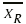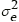% CV Ñ
Cmax 1.65444 0.83225 55.14 217.63
ln (Cmax) 0.39249 0.48509 79.01 1.92
AUC(0-8) 5.23111 5.99238 46.80 256.41
ln (AUC(0-8)) 1.52090 0.30899 60.17 2.41

Table 8

Comments on the results obtained by (Chow and Liu, 2000) concerning the analysis of variance of the log-transformed data for AUC(0-8)

(Chow and Liu, 2000) concluded the following statement: “The estimated CV is about 36.55%, which is high, but not unusual in bioavailability and bioequivalence studies. The index of sensitivity defined in (Equation 5.3.10) is estimated to be about 3.28. Therefore, as discussed in (section 5.3.2), this study has little power to conclude bioequivalence if (Schuirmann, 1987) two one sided test procedures and the ± 20% rule are applied.”

(Chow and Liu, 2000) assumed that the results obtained from the analysis of variance for log-transformed data are as the results obtained from the analysis of variance of raw data. Accordingly, they computed the intrasubject coefficient of variation (CV) and the measure of sensitivity using (Equations 1 and 4):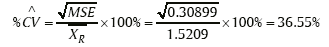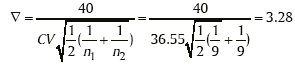On the other hand, different results for CV and ∇ are obtained using (Equations 2 and 5):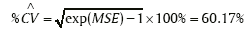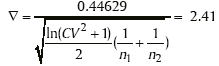Also in (page 195), (Chow and Liu, 2000) gave summary of points and interval estimation of intrasubject variability for data sets from the Analysis of Variance of ln (AUC(0-8)). That is; for the parameter, the point estimate = 0.3090 and 90% confidence interval foris [Le, Ue] = [0.17, 0.72] if one interested to find the 95% confidence interval for the parameter CV, the point estimation of CV = 60.17%, then according to (Equation 3), the 95% confidence interval for CV is: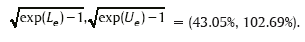#### Discussion

In this note, we try to clarify the confusion in finding the intrasubject coefficient of variation (CV) based on the additive model (raw data model) and the multiplicative model (log-transformed model). Since the information on intrasubject coefficient of variation is still needed for sample size planning in future studies, one must specify the model (raw data or log-transformed data) in the crossover design and then choose the suitable equation for the calculation of coefficient of variation (CV).

#### References

Select your language of interest to view the total content in your interested language

### Article Usage

• Total views: 18055
• [From(publication date):
August-2010 - Jul 16, 2019]
• Breakdown by view type
• HTML page views : 13754Can't read the image? click here to refresh